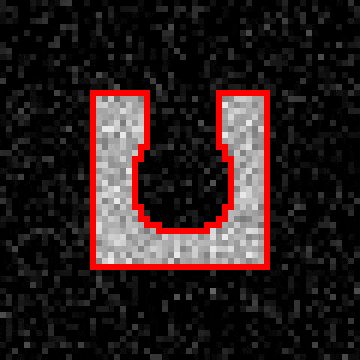# Filling a cavity with a level set method¶

This example shows how to fill a concave cavity using a level set method.Edge of segmented region shown as a red outline on top of the original image. The animation shows evolution of the edge as a function of iteration number.

def levelset_fill_cavity():
"""
Demonstrates how to fill a non-convex cavity using a level-set method.
"""

# Create an image that contains a rectangle with a cavity in its edge.
# For this example we make a 2D image for easier visualization,
# but everything should work the same for a 3D volume image, too.
c = 30 # Radius of the image
geom = pi.newimage(ImageDataType.UINT8, 2 * c, 2 * c)
pi.box(geom, [c - 15, c - 15, 0], 30, 128)      # The box
pi.sphere(geom, [c, c, 0], 8, 0)                # Part of cavity
pi.sphere(geom, [c, c - 6, 0], 6, 0)            # Part of cavity
pi.sphere(geom, [c, c - 12, 0], 6, 0)           # Part of cavity

# Noise to make the image more realistic
pi.noise(geom, 0, 20)

# Save the geometry
pi.writetif(geom, output_file('levelset_geom'))

# Initialize an image that holds the level set function.
# After iteration below, the segmentation is the part of phi
# that has positive value.
phi = pi.newlike(geom, ImageDataType.FLOAT32)

# Update phi iteratively
for n in np.arange(0, 200):
print(f"Iteration {n}" )

# Construct force field that acts on the surface defined by phi
# The force will be the sum of three terms.
F = pi.newlike(phi)

# First term: the force is positive inside the object and negative everywhere else.
# This makes the surface take the shape of the object.
pi.copy(geom, F)
pi.divide(F, 128)
pi.subtract(F, 0.5)

# Second term: Penalize high curvature by making curvy
# regions less curved.
kappa = pi.newlike(phi)
pi.meancurvature(phi, kappa, 0.5)

# Multiply the curvature values to scale them correctly.
pi.multiply(kappa, -5)

# Remove negative curvature values. They correspond to
# convex shapes, and we want to zero those so that
# they don't have any effect on the surface.
pi.max(kappa, 0)

# Add kappa to the force term

# Third term: Normal force
# This term makes the surface move towards its normal.
# This term is not required in this example.
#L = pi.newlike(phi)
#pi.multiply(L, 0.01)

# Smooth the total force a little bit
# This is not strictly by the book, but smoothing seems to
# make phi converge faster to a smoother result.
tmp = pi.newlike(F)
pi.gaussfilter(F, tmp, 0.5, BoundaryCondition.NEAREST)
pi.set(F, tmp)

# Multiply by time step
dt = 1
pi.multiply(F, dt)

# Add force*dt to the surface